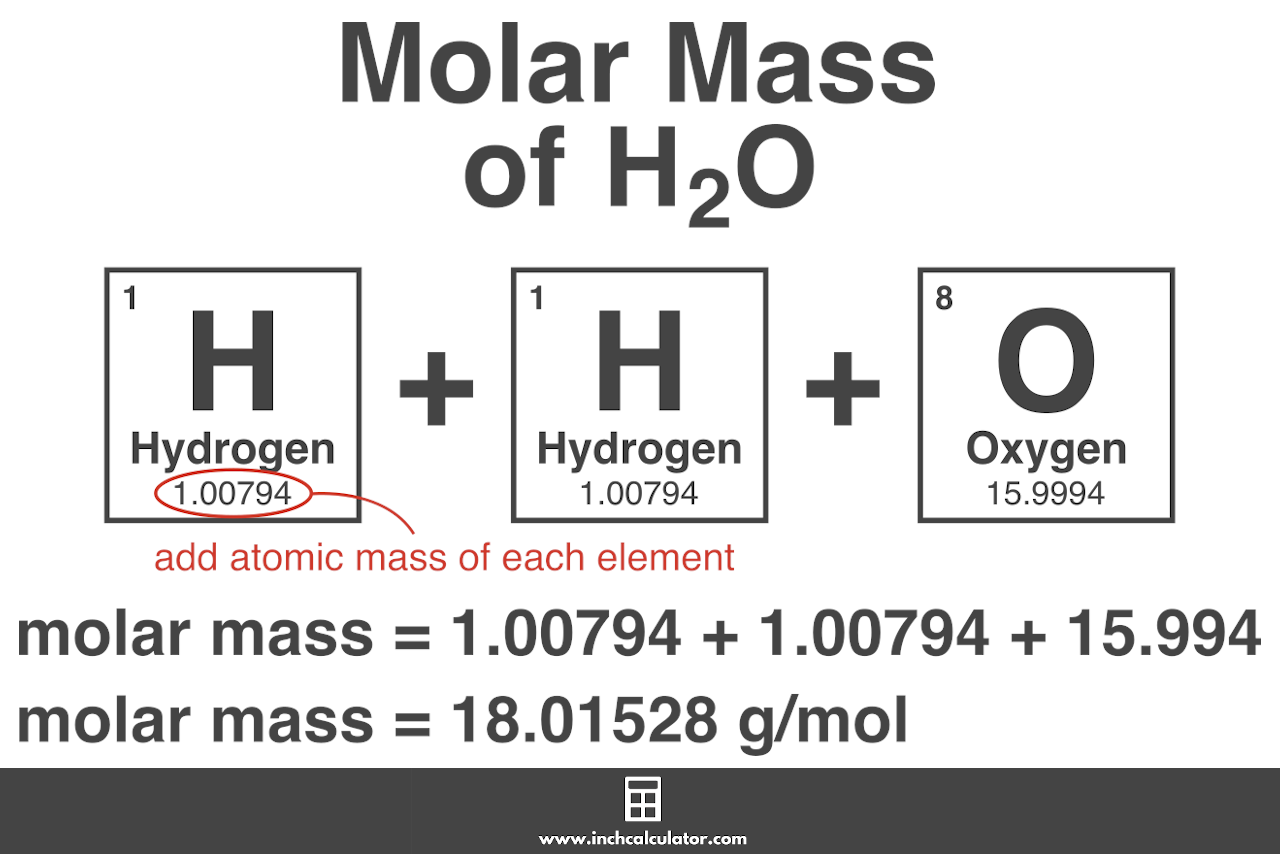# Molar Mass Calculator

Calculate the molar mass of a compound or element by selecting it from the lists or entering the chemical formula below.

## Molar Mass:

g/mol
Learn how we calculated this below

## What is Molar Mass?

Molar mass is the mass of a compound in grams per mole of a substance, and it’s expressed in g/mol. Molar mass, sometimes referred to as molecular weight or molecular mass, is equal to the sum of the atomic masses of each element in the compound.

The atomic mass is the mass of 1 mol of an atom – so if you add up the atomic masses of all the atoms in the compound, the sum is the molar mass or molecular weight of that compound expressed in g/mol.

A mole is used as a standard measurement for a very large quantity of very small units of matter. One mole is equal to 6.02214076 × 1023 elementary entities, such as atoms, molecules, electrons, or ions. This numerical value is known as Avogadro’s number.

Another unit often used to describe molecular weight or molar mass is the Dalton (Da). A Dalton is similar to g/mol but is used for more precise measurements of atoms and subatomic particles in chemistry and physics. For most practical purposes, a Dalton can be considered equivalent to g/mol.

### How is Molar Mass Used?

Molar mass is used to convert between the weight of a substance and the number of moles of that substance in chemistry. Chemical reactions are normally described in terms of moles of reactants and moles of products.

However, the reactants and products are frequently measured in grams or liters. The reactants must be weighed out in the correct amounts based on the number of moles required.

Then the product of the reaction is weighed to calculate the yield of the reaction in grams, which can be converted to moles. Each of these steps uses the molar mass as a conversion factor between grams and moles.

## How to Calculate Molar Mass

You can calculate the molecular weight of the compound by adding the atomic masses of each element in the compound using the periodic table. The National Institute of Standards and Technology published the atomic weights for each element and is a good resource to consult.

For example, let’s calculate the molar mass of water (H2O).

H2O is composed of two hydrogen (H) atoms and one oxygen (O) atom.

The atomic mass of hydrogen is 1.00794 and oxygen is 15.9994. The formula to calculate the molecular weight based on the atomic weights looks like this:

molar mass = 1.00794 (H) + 1.00794 (H) + 15.9994 (O)

Therefore, the molar mass of H2O is 18.01528 g/mol.### Molecular Weight Formula

Another way to calculate molecular weight is by using the molar mass formula. To use the formula, you’ll need the total mass of the substance in grams and the quantity in moles.

M = m / n

Thus, the molar mass M is equal to the mass of substance m in grams divided by the quantity n in moles.

Of course, the calculator above is the easiest way to find the molar mass of an element or compound.

## References

1. LibreTexts, Molar Mass, 2020, August 13, https://chem.libretexts.org/@go/page/79550
2. National Institute of Standards and Technology | Physical Measurements Laboratory, Atomic Weights and Isotopic Compositions for All Elements, https://physics.nist.gov/cgi-bin/Compositions/stand_alone.pl
3. National Institute of Standards and Technology, NIST Guide to the SI, Chapter 8, https://www.nist.gov/pml/special-publication-811/nist-guide-si-chapter-8